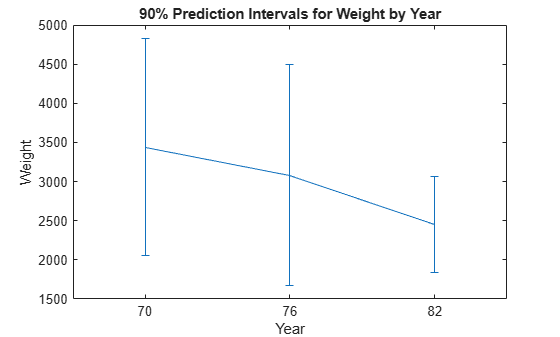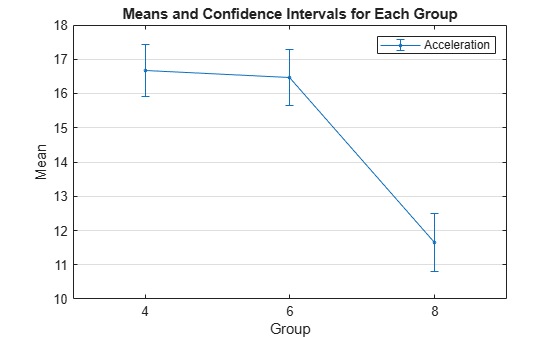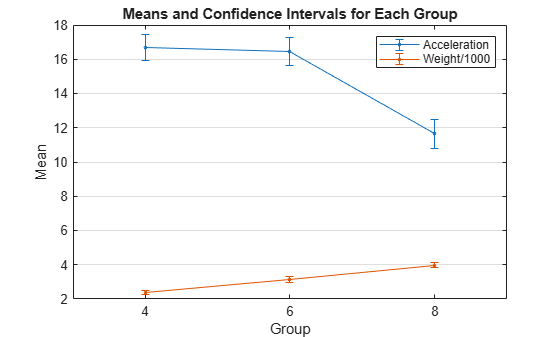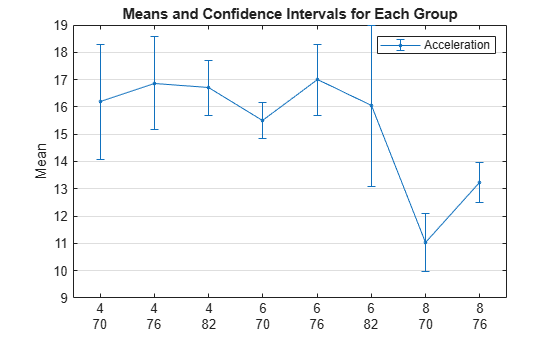# grpstats

Summary statistics organized by group

## Syntax

``tblstats = grpstats(tbl,groupvars)``
``tblstats = grpstats(tbl,groupvars,whichstats)``
``tblstats = grpstats(tbl,groupvars,whichstats,Name,Value)``
``stats = grpstats(X,group)``
``[stats1,...,statsN] = grpstats(X,group,whichstats)``
``[stats1,...,statsN] = grpstats(X,group,whichstats,"Alpha",a)``
``grpstats(X,group,alpha)``

## Description

````tblstats = grpstats(tbl,groupvars)` returns a table with group summary statistics for the variables in the table `tbl`, where the function determines the groups according to the grouping variables in `tbl` specified by `groupvars`.If all variables in `tbl` (other than the grouping variables) are numeric or logical, then the summary statistic is the mean of each group for each variable in `tbl`. Otherwise, the summary statistic is the number of elements in each group. `tblstats` contains a row for each observed unique value or combination of values in the grouping variables.```
````tblstats = grpstats(tbl,groupvars,whichstats)` specifies the summary statistic types `whichstats`.```

example

````tblstats = grpstats(tbl,groupvars,whichstats,Name,Value)` specifies additional options using one or more name-value arguments. For example, `"DataVars",[2,4]` instructs the function to compute summary statistics for the second and fourth variables in `tbl`.```

example

````stats = grpstats(X,group)` returns an array with group summary statistics for the columns of the matrix `X`, where the function determines the groups by the grouping variables in `group`.If `X` is a numeric or logical matrix, then the summary statistic is the mean of each group for each column of `X`. Otherwise, the summary statistic is the number of elements in each group. `stats` contains a row for each observed unique combination of the grouping variables.```

example

````[stats1,...,statsN] = grpstats(X,group,whichstats)` specifies the summary statistic types `whichstats` and returns an array for each summary statistic.```

example

````[stats1,...,statsN] = grpstats(X,group,whichstats,"Alpha",a)` also specifies the significance level `a` for confidence and prediction intervals.```

example

````grpstats(X,group,alpha)` plots the group means of data in the numeric or logical matrix `X`, grouped by the variables in `group`. The function also plots the 100×(1 – `alpha`)% confidence interval for each group mean. The grouping variable values are on the horizontal plot axis.If `X` is a matrix, then `grpstats` plots the means and confidence intervals for each column of `X`.If `group` is a cell array of grouping variables, then `grpstats` plots the means and confidence intervals for the groups determined by the observed unique combinations of the grouping variables.```

## Examples

collapse all

Compute summary statistics for input data in a table. Group the input data using one or two grouping variables, and specify one or two types of summary statistics to compute.

Load the `patients` data set.

`load patients`

Create a table that contains the variables `Gender`, `Age`, `Weight`, and `Smoker`.

`tbl = table(Gender,Age,Weight,Smoker);`

`Gender` is a cell array with the two unique values `Male` and `Female`. The variables `Age` and `Weight` have numeric values, and `Smoker` has logical values.

Compute the mean for the numeric and logical arrays in `tbl` grouped by `Gender`.

`tblstats1 = grpstats(tbl,"Gender")`
```tblstats1=2×5 table Gender GroupCount mean_Age mean_Weight mean_Smoker __________ __________ ________ ___________ ___________ Male {'Male' } 47 38.915 180.53 0.44681 Female {'Female'} 53 37.717 130.47 0.24528 ```

`tblstats1` is a table with two rows corresponding to the unique values in `Gender`. The `GroupCount` column shows the number of observations in each group. The columns `mean_Age`, `mean_Weight`, and `mean_Smoker` show the means of `Age`, `Weight`, and `Smoker` grouped by `Gender`.

Compute the mean for `Age` and `Weight` grouped by the values in `Smoker`. Specify `Age` and `Weight` as the variables for which you want to compute summary statistics by using the `DataVars` name-value argument. You must use `DataVars` because the input `tbl` includes the `Gender` variable, which is a cell array, and the built-in summary statistic `mean` is valid only for numeric and logical arrays.

`tblstats2 = grpstats(tbl,"Smoker","mean","DataVars",["Age","Weight"])`
```tblstats2=2×4 table Smoker GroupCount mean_Age mean_Weight ______ __________ ________ ___________ 0 false 66 37.97 149.91 1 true 34 38.882 161.94 ```

Compute the minimum and maximum weight grouped by the combinations of values for `Gender` and `Smoker`.

```tblstats3 = grpstats(tbl,["Gender","Smoker"],["min","max"], ... "DataVars","Weight")```
```tblstats3=4×5 table Gender Smoker GroupCount min_Weight max_Weight __________ ______ __________ __________ __________ Male_0 {'Male' } false 26 158 194 Male_1 {'Male' } true 21 164 202 Female_0 {'Female'} false 40 111 147 Female_1 {'Female'} true 13 115 146 ```

`Smoker` and `Gender` each have two unique values, so the output table includes four rows for the possible combinations: Male Nonsmoker (`Male_0`), Male Smoker (`Male_1`), Female Nonsmoker (`Female_0`), and Female Smoker (`Female_1`).

Specify the names for the columns in the output by using the `VarNames` name-value argument.

```tblstats4 = grpstats(tbl,["Gender","Smoker"],["min","max"], ... "DataVars","Weight", ... "VarNames",["Gender","Smoker","Group Count", ... "Lowest Weight","Highest Weight"])```
```tblstats4=4×5 table Gender Smoker Group Count Lowest Weight Highest Weight __________ ______ ___________ _____________ ______________ Male_0 {'Male' } false 26 158 194 Male_1 {'Male' } true 21 164 202 Female_0 {'Female'} false 40 111 147 Female_1 {'Female'} true 13 115 146 ```

Compute group means for input data in a matrix. Group the input data using one or two grouping variables.

Load the `carsmall` data set, which contains measurements of 100 cars.

`load carsmall`

Compute group means for the variable `Acceleration` grouped by the variables `Origin` and `Cylinders`. The variable `Acceleration` is the time from 0 to 60 MPH in seconds. The grouping variable `Origin` is the country of origin for each car (France, Germany, Italy, Japan, Sweden, or USA). The grouping variable `Cylinders` has three unique values, `4`, `6`, and `8`, indicating the number of cylinders in each car.

Calculate the mean acceleration grouped by the country of origin.

`means = grpstats(Acceleration,Origin)`
```means = 6×1 14.4377 18.0500 15.8867 16.3778 16.6000 15.5000 ```

`means` is a 6-by-1 vector of mean accelerations, where each value corresponds to a country of origin.

Calculate the mean acceleration grouped by both the country of origin and number of cylinders. Return the group names along with the mean acceleration for each group.

```[means,grps] = grpstats(Acceleration,{Origin,Cylinders}, ... ["mean","gname"])```
```means = 10×1 17.0818 16.5267 11.6406 18.0500 15.9143 15.5000 16.3375 16.7000 16.6000 15.5000 ```
```grps = 10x2 cell {'USA' } {'4'} {'USA' } {'6'} {'USA' } {'8'} {'France' } {'4'} {'Japan' } {'4'} {'Japan' } {'6'} {'Germany'} {'4'} {'Germany'} {'6'} {'Sweden' } {'4'} {'Italy' } {'4'} ```

The two grouping variables `Origin` and `Cylinders` have 18 possible combinations because `Origin` has six unique values and `Cylinders` has three unique values. Only 10 of the possible combinations appear in the data, so `means` is a 10-by-1 vector of group means corresponding to the observed combinations of values. The output `grps` shows the 10 observed combinations of grouping variable values. For example, the mean acceleration of 4-cylinder cars made in France is 18.05.

Compute multiple group summary statistics for input data in a matrix.

Load the `carsmall` data set, which contains measurements of 100 cars.

`load carsmall`

Compute group summary statistics for the variable `Acceleration` grouped by the variable `Origin`. The variable `Acceleration` is the time from 0 to 60 MPH in seconds, and the grouping variable `Origin` is the country of origin for each car (France, Germany, Italy, Japan, Sweden, or USA).

Return the minimum and maximum acceleration grouped by the country of origin.

```[grpMin,grpMax,grp] = grpstats(Acceleration,Origin, ... ["min","max","gname"])```
```grpMin = 6×1 8.0000 15.3000 13.9000 12.2000 15.7000 15.5000 ```
```grpMax = 6×1 22.2000 21.9000 18.2000 24.6000 17.5000 15.5000 ```
```grp = 6x1 cell {'USA' } {'France' } {'Japan' } {'Germany'} {'Sweden' } {'Italy' } ```

The car with the lowest acceleration is made in the USA, and the car with the highest acceleration is made in Germany.

Compute summary statistics for input data in a table. Pass in `[]` for the grouping variable so that `grpstats` computes summary statistics without grouping.

Load the `patients` data set.

`load patients`

Create a table that contains the variables `Age`, `Weight`, and `Smoker`.

`tbl = table(Age,Weight,Smoker);`

The variables `Age` and `Weight` have numeric values, and `Smoker` has logical values.

Compute the mean, minimum, and maximum for the numeric arrays `Age` and `Weight` and the logical array `Smoker`, with no grouping.

`tblstats = grpstats(tbl,[],["mean","min","max"])`
```tblstats=1×10 table GroupCount mean_Age min_Age max_Age mean_Weight min_Weight max_Weight mean_Smoker min_Smoker max_Smoker __________ ________ _______ _______ ___________ __________ __________ ___________ __________ __________ All 100 38.28 25 50 154 111 202 0.34 false true ```

The observation name `All` indicates that `grpstats` uses all observations in `tbl` to compute the summary statistics.

Compute and plot means and prediction intervals for each group of input data in a matrix.

Load the `carsmall` data set, which contains measurements of 100 cars.

`load carsmall`

Compute group summary statistics for the variable `Weight` grouped by the variable `Model_Year`. The variable `Weight` contains car weight values, and the grouping variable `Model_Year` has three unique values, `70`, `76`, and `82`, which correspond to the model years 1970, 1976, and 1982.

Calculate the mean weight and 90% prediction intervals for each model year.

```[means,pred,grp] = grpstats(Weight,Model_Year, ... ["mean","predci","gname"],"Alpha",0.1);```

Plot error bars showing the mean weight and 90% prediction intervals grouped by model year. Specify the horizontal tick labels as the group names.

```f = figure; ngrps = length(grp); % Number of groups errorbar((1:ngrps)',means,pred(:,2)-means) xlim([0.5 3.5]) f.CurrentAxes.XTick = 1:ngrps; f.CurrentAxes.XTickLabel = grp; title("90% Prediction Intervals for Weight by Year") xlabel("Year") ylabel("Weight")```Plot group means and confidence intervals for input data in a matrix. Group the input data using one or two grouping variables, and specify one or two variables for which you want to plot the summary statistics.

Load the `carsmall` data set, which contains measurements of 100 cars.

`load carsmall`

The variable `Acceleration` is the time from 0 to 60 MPH in seconds. The grouping variable `Cylinders` is the number of cylinders in each car.

Plot the mean acceleration grouped by cylinder, with 95% confidence intervals.

```grpstats(Acceleration,Cylinders,0.05); legend("Acceleration")```The mean acceleration for cars with 8 cylinders is significantly lower than for cars with 4 or 6 cylinders.

The variable `Weight` is the weight value for each car. Plot the mean acceleration and weight grouped by cylinder, with 95% confidence intervals. Scale the `Weight` values by 1000 so the means of `Weight` and `Acceleration` are the same order of magnitude.

```grpstats([Acceleration,Weight/1000],Cylinders,0.05); legend("Acceleration","Weight/1000")```The mean weight of cars increases with the number of cylinders, and the mean acceleration decreases with the number of cylinders.

The `Model_Year` variable has three unique values, 70, 76, and 82, which correspond to the model years 1970, 1976, and 1982. Plot the mean acceleration grouped by both cylinder and model year. Specify 95% confidence intervals.

```grpstats(Acceleration,{Cylinders,Model_Year},0.05); legend("Acceleration")```The two grouping variables `Cylinders` and `Model_Year` have nine possible combinations of values, because each variable has three unique values. The plot does not show 8-cylinder cars with the model year 1982 because the data does not include this combination.

The mean acceleration of 8-cylinder cars made in 1976 is significantly larger than the mean acceleration of 8-cylinder cars made in 1970.

Define a custom summary statistic by using an anonymous function. Pass the anonymous function to `grpstats` to compute the custom summary statistic for each group of input data.

Load the `patients` data set.

`load patients`

Create a table that contains the variables `Age`, `Smoker`, and `LastName`.

`tbl = table(Age,Smoker,LastName);`

Find the number of smokers for each age group by using a custom function that computes the sum of each column of an input matrix.

```f_sum = @(x)sum(x,1); tblstats1 = grpstats(tbl,"Age",f_sum,"DataVars","Smoker", ... "VarNames",["Age","Group Count","Number of Smokers"])```
```tblstats1=25×3 table Age Group Count Number of Smokers ___ ___________ _________________ 25 25 6 1 27 27 1 1 28 28 5 2 29 29 3 0 30 30 4 1 31 31 4 2 32 32 4 1 33 33 3 3 34 34 1 0 35 35 2 0 36 36 4 0 37 37 5 2 38 38 6 2 39 39 8 3 40 40 4 1 41 41 3 0 ⋮ ```

`tblstats1` is a table with 25 rows corresponding to the unique values in `Age`. The `Group Count` column shows the number of observations in each age group, and the last column shows the number of smokers in each group.

Determine the mean length of the last name for each age group by using a custom function that computes the mean length of the elements in a cell array.

```f_length = @(x)mean(cellfun("length",x)); tblstats2 = grpstats(tbl,"Age",f_length,"DataVars","LastName", ... "VarNames",["Age","Group Count","Mean Length of Last Name"])```
```tblstats2=25×3 table Age Group Count Mean Length of Last Name ___ ___________ ________________________ 25 25 6 5.6667 27 27 1 6 28 28 5 5.4 29 29 3 5.6667 30 30 4 6.5 31 31 4 5.25 32 32 4 6.5 33 33 3 6.3333 34 34 1 9 35 35 2 7.5 36 36 4 6.25 37 37 5 8.2 38 38 6 5.8333 39 39 8 6.125 40 40 4 5.5 41 41 3 5.3333 ⋮ ```

## Input Arguments

collapse all

Input data, specified as a table. `tbl` must include at least one grouping variable, which you specify using `groupvars`. You can select variables for which to calculate summary statistics by using the `DataVars` name-value argument.

Each variable in `tbl` can be a numeric, logical, categorical, datetime, duration, or calendar duration vector, a character or string array, or a cell array of character vectors. You cannot specify a calendar duration vector as a grouping variable.

Identifiers for the grouping variables in the table input `tbl`, specified as one of the values in this table.

ValueDescription
Character vector, string array, or cell array of character vectorsNames of the grouping variables
Vector of positive integersVariable numbers of the grouping variables
Vector of logical values with the number of elements equal to the number of variables in `tbl`Logical indicator with the value `true` for grouping variables and `false` otherwise
`[]`No groups (returns summary statistics for all data)

The variables specified by `groupvars` as grouping variables must have a data type that is valid for grouping variables: numeric, logical, categorical, datetime, or duration vector; character or string array; or cell array of character vectors.

For example, consider an input table `tbl` with six variables. The fourth variable is named `Gender`. To specify the variable `Gender` as the grouping variable, you can use one of these syntaxes:

• `tblstats = grpstats(tbl,"Gender")`

• `tblstats = grpstats(tbl,4)`

• ```tblstats = grpstats(tbl,logical([0 0 0 1 0 0]))```

Data Types: `single` | `double` | `logical` | `char` | `string` | `cell`

Types of summary statistics to compute, specified as one of the following values.

• Character vector or string scalar specifying the built-in summary statistic, as described in this table.

Built-in Summary StatisticDescription
`"gname"`Group name
`"numel"`Count, or number, of non-`NaN` elements

If you specify input data as a table `tbl`, then the output table `tblstats` includes the group name and group count by default. You do not need to specify `"gname"` and `"numel"`.

For numeric and logical variables, you can also specify one of these built-in summary statistics.

Built-in Summary StatisticDescription
`"mean"`Mean
`"sem"`Standard error of the mean
`"std"`Standard deviation
`"var"`Variance
`"min"`Minimum
`"max"`Maximum
`"range"`Range
`"meanci"`95% confidence interval for the mean. You can specify different significance levels using the `Alpha` name-value argument.
`"predci"`95% prediction interval for a new observation. You can specify different significance levels using the `Alpha` name-value argument.

• Function handle to specify any other types of summary statistics. You can use the handle to any function that accepts a column or matrix of data, and returns the same size output each time `grpstats` calls the function handle (even if the output for some groups is empty).

• If the function accepts a column of data, then the function can return either a scalar value or an nvals-by-1 column vector for descriptive statistics of length nvals (for example, a confidence interval has length two). If the function accepts a matrix, the function must return either a 1-by-ncols row vector or an nvals-by-ncols matrix, where ncols is the number of columns in the input data matrix.

• For functions that do not compute column-wise statistics, specify the computation direction while specifying the function. For example, to use the `sum` function, specify the function handle as `@(x)sum(x,1)` because `sum` computes column-wise statistics for matrices with two or more rows, but not for single-row matrices.

• String array or a cell array of character vectors or function handles to specify multiple types of summary statistics.

Example: `stat1 = grpstats(X,group,"sem")`

Example: ```stat1 = grpstats(X,group,@(x)sum(x,1))```

Example: ```[stat1,stat2,stat3] = grpstats(X,group,{"mean","std",@skewness})```

Input data, specified as a vector or matrix. If `X` is a matrix, then `grpstats` returns summary statistics for each column of `X`.

Data Types: `single` | `double` | `logical` | `char` | `string` | `cell` | `categorical` | `datetime` | `duration` | `calendarDuration`

Grouping variables for the input array `X`, specified as a numeric, logical, categorical, datetime, or duration vector, a character or string array, a cell array of character vectors, or a cell array of multiple grouping variables.

`grpstats` groups data in `X` using the grouping variable values. Use `[]` to compute summary statistics for all data, without grouping.

You can also use more than one grouping variable to group data for summary statistics. In this case, specify a cell array of grouping variables.

For example, consider the two grouping variables `Gender` and `Smoker`. The variable `Gender` is a string array with the values `"Male"` and `"Female"`, and the variable `Smoker` is a logical vector with the value `0` for nonsmokers and `1` for smokers. If you specify the cell array `{Gender,Smoker}`, then `grpstats` divides observations into four groups: Male Smoker, Male Nonsmoker, Female Smoker, and Female Nonsmoker. `grpstats` returns summary statistics only for the combinations of values that exist in the grouping variables (not all possible combinations).

Data Types: `single` | `double` | `logical` | `char` | `string` | `cell` | `categorical` | `datetime` | `duration`

Significance level for plotting, specified as a scalar value in the range (0,1).

Use the syntax `grpstats(X,group,alpha)` to plot group means and corresponding 100×(1 – `alpha`)% confidence intervals.

Data Types: `double`

### Name-Value Arguments

Specify optional pairs of arguments as `Name1=Value1,...,NameN=ValueN`, where `Name` is the argument name and `Value` is the corresponding value. Name-value arguments must appear after other arguments, but the order of the pairs does not matter.

Before R2021a, use commas to separate each name and value, and enclose `Name` in quotes.

Example: `"DataVars",[1,3,4],"Alpha",0.01` calculates summary statistics for the 1st, 3rd, and 4th variables in the input table, with 99% confidence intervals.

Significance level for confidence and prediction intervals, specified as a scalar value in the range (0,1).

When you include `"meanci"` or `"predci"` in `whichstats`, you can use `Alpha` to specify the significance level for confidence or prediction intervals, respectively. If you specify the value α, then `grpstats` returns 100×(1 – α)% confidence or prediction intervals. If you do not specify a value for `Alpha`, then `grpstats` returns 95% intervals (α = 0.05).

Example: `"Alpha",0.1`

Data Types: `double`

Table variables in `tbl` for which to compute summary statistics, specified as one of the values in this table.

ValueDescription
Character vector, string array, or cell array of character vectorsNames of the table variables
Vector of positive integersVariable numbers of the table variables in `tbl`
Vector of logical values with the number of elements equal to the number of variables in `tbl`Logical indicator with the value `true` to include the table variables and `false` otherwise

Example: `"DataVars",["Height","Weight"]`

Data Types: `double` | `string` | `cell` | `char`

Variable (column) names for the output table `tblstats`, specified as a string array or a cell array of character vectors. By default, `grpstats` constructs output variable names by appending a prefix to them from the input data `tbl`. This prefix corresponds to the summary statistic name.

Example: `"VarNames",["Gender","GroupCount","MaleMean","FemaleMean"]`

Data Types: `string` | `cell`

## Output Arguments

collapse all

Group summary statistics for the table input `tbl`, returned as a table.

`tblstats` contains a row for each observed unique value or combination of values in the grouping variables, and includes columns for the following:

• All grouping variables specified by `groupvars`

• The variable `GroupCount`, which contains the number of observations in each group

• Group summary statistic values for all variables in `tbl` (other than the grouping variables) or for only the variables specified by `DataVars`

The total number of columns in `tblstats` is ngroupvars + 1 + ndatavars×nstats, where ngroupvars is the number of observed unique values or combinations of values in `groupvars`, ndatavars is the number of variables for which summary statistics are computed, and nstats is the number of summary statistic types specified in `whichstats`.

`grpstats` assigns default names to the columns in `tblstats` unless you specify column names using the name-value argument `VarNames`.

Group summary statistic values for the matrix input `X`, returned as an ngroups-by-ncols array. Here, ngroups is the number of observed unique values or combinations of values in the grouping variables specified in `group`, and ncols is the number of columns in `X`. Each column of `stats` contains the summary statistics for the corresponding column of `X`.

If `X` is a numeric or logical matrix, then the summary statistic is the mean of each group. Otherwise, the summary statistic is the number of elements in each group.

Multiple group summary statistics for the matrix input `X`, returned as ngroups-by-ncols arrays. Here, ngroups is the number of observed unique values or combinations of values in the grouping variables specified in `group`, and ncols is the number of columns in `X`. Each column of the output array contains the summary statistics for the corresponding column of `X`.

You must specify an output argument for each type of summary statistic specified in `whichstats`.

If a summary statistic type in `whichstats` returns a value of length nvals (for example, a confidence interval is a descriptive statistic of length two), then the corresponding output argument is an ngroups-by-ncols-by-nvals array.

## Algorithms

• `grpstats` computes summary statistic values for each observed unique value or combination of values in the grouping variables.

• If you specify a single grouping variable, then the output of `grpstats` contains a row for each observed unique value of the grouping variable. `grpstats` sorts the groups by order of appearance (if the grouping variable is a character vector or string scalar); in ascending numeric order (if the grouping variable is numeric); or in order of by category (if the grouping variable is categorical).

• If you specify multiple grouping variables, then the output of `grpstats` contains a row for each observed unique combination of values in the grouping variables. For example, if you specify two grouping variables, each with two values, then the output has four possible combinations of grouping variable values. The function computes summary statistics only for the observed combinations that exist in the input grouping variables (not all possible combinations). `grpstats` sorts the groups by the values of the first grouping variable, then the second grouping variable, and so on.

• `grpstats` ignores missing values in `tbl`, `X`, and `group`. Missing values depend on the data type:

• `NaN` for `double`, `single`, `duration`, and `calendarDuration`

• `NaT` for `datetime`

• `<missing>` for `string`

• `<undefined>` for `categorical`

• `' '` for `char`

• `{''}` for `cell` of character vectors

## Alternative Functionality

MATLAB® includes the function `groupsummary`, which also returns group summaries and is recommended when you are working with a table. `groupsummary` allows you to specify whether to include groups that consist of missing values and groups with zero elements in the output. Also, the function supports various group binning schemes and anonymous functions that require more than one input argument for custom summary statistics.

## Version History

Introduced before R2006a